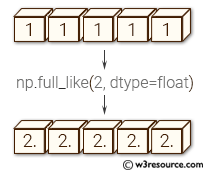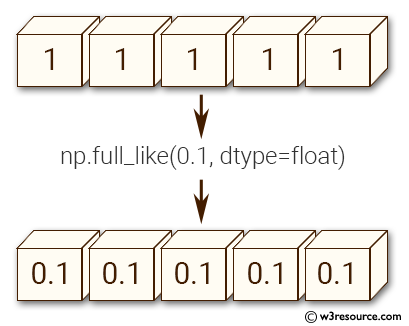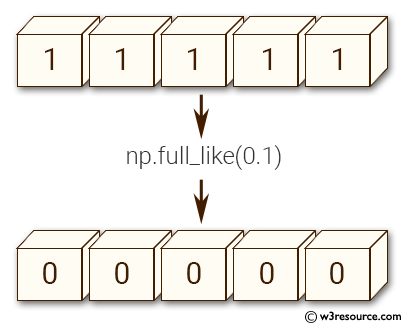# NumPy array creation: full_like() function

## full_like() function

The full_like() function return a full array with the same shape and type as a given array.

Syntax:

`numpy.full_like(a, fill_value, dtype=None, order='K', subok=True)`Version: 1.15.0

Parameter:

Name Description Required /
Optional
a The shape and data-type of a define these same attributes of the returned array. Required
fill_value Fill value. Required
dtype Overrides the data type of the result. optional
order Overrides the memory layout of the result. 'C' means C-order, 'F' means F-order, 'A' means 'F' if a is Fortran contiguous, 'C' otherwise. 'K' means match the layout of a as closely as possible. optional
subok If True, then the newly created array will use the sub-class type of 'a', otherwise it will be a base-class array. Defaults to True. optional

Return value:

[ndarray] Array of fill_value with the same shape and type as a.

Example: numpy.full_like() function

``````>>> import numpy as np
>>> a = np.arange(5, dtype=int)
>>> np.full_like(a, 1)
array([1, 1, 1, 1, 1])
>>> np.full_like(a, 0.1)
array([0, 0, 0, 0, 0])
>>> np.full_like(a, 0.1, dtype=float)
array([ 0.1,  0.1,  0.1,  0.1,  0.1])
>>> np.full_like(a, np.nan, dtype=np.double)
array([ nan,  nan,  nan,  nan,  nan])
``````

Pictorial Presentation:Example: numpy.full_like() function

``````>>> import numpy as np
>>> b = np.arange(5, dtype=np.double)
>>> np.full_like(b, 0.5)
array([ 0.5,  0.5,  0.5,  0.5,  0.5])
``````

Python - NumPy Code Editor:

Previous: full()
Next: From existing data array()

﻿